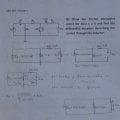# Question regarding Norton Equivalent Circuits and Inductor current.

#### Robert Murch

Joined Nov 2, 2015
43
Hello everyone reading thanks for taking the time to try and help me. I have uploaded and image of the question and the work I have done so far:The problem I am having is coming up with the differential equation that describes the current through the inductor. I know that for a capacitor and a Thevinan equivalent circuit I would simply use kvl and then do some substitutions. However, for this Norton equivalent circuit with and inductor, I cannot seem to figure out how to come up with an equation describing Il2 (as labled). I tried kcl at the top node(Vx): -In +3/Vx + Il2 = 0 but then I couldn't describe the voltage Vx. I also tried two current loops, first loop: 3(In - Il2) = 0, second loop: 3(Il2 - In) = 0. However that seems to suggest that Il2 = In which does not seem right. Can someone tell me where I went wrong or what I need to do after this step if I have done everything correctly so far?

#### WBahn

Joined Mar 31, 2012
29,521
One of the problems is that you ended up with Is (the original current source) as your Norton equivalent current source. Does that make sense? What was the point of finding Isc just above it?

From the statement in your post, "I tried kcl at the top node(Vx): -In +3/Vx + Il2 = 0," if you would track your units you would see that this is guaranteed not to work. The first and third terms are currents while the middle term, 3/Vx, should be 3Ω/Vx, which will have units of 1/ampere.

#### Robert Murch

Joined Nov 2, 2015
43
Okay I seem to have left it as 10sin(2t) when I meant (10/3)sin(2t) which is Isc. Using -In +3/Vx +Il(t) = 0 can i not just substitute Vx as L(dIl/dt)? I did that and came up with (10/3)sin(2t) = (1/3)L((d/dt)(asin(2t)+bcos(2t))+asin(2t)+bcos(2t). Where the forcing function Il(t) = asin(2t)+bcos(2t).

#### WBahn

Joined Mar 31, 2012
29,521
Look carefully at : -In +3/Vx +Il(t) = 0 (which should be -In +3Ω/Vx +Il(t) = 0)

What does the first term, -In, represent? A current, yes?

What does the third term, +Il(t), represent? A current, yes?

What does the second term, +3Ω/Vx, represent? Hint: It is NOT a current!

But, in order to add them altogether, they MUST have the same units -- you can't add gallons and feet.